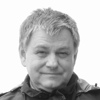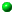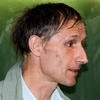Oleg Viro's home page

texts for studentsElementary Topology. Textbook in Problems,
by O.Ya.Viro, O.A.Ivanov, V.M.Kharlamov and N.Y.Netsvetaev.Configurations of Skew Lines in the 3-Dimensional Space
by J.Viro and O.Viro
This is about links made of lines. One has to require that the lines are not only disjoint, but also non-parallel. This gives the lines an ability to be linked with each other. As long as the number of lines is not large (less than 6), the linking numbers rule. Then the Jones polynomial comes. On a horizont the Khovanov homology can be recognized...Introductions into Topology of Real Algebraic VarietiesIntroductions to patchworkingDequantization of real algebraic geometry on logarithmic paper
A talk by Oleg Viro at the Third European Congress of Mathematicians (Barcelona, 2000). It bridged patchworking and Litvinov - Maslov dequantization of positive real numbers and started up a broad development of Tropical geometry.What is an Amoeba?   Notices AMS, 49:8 (2002), 916-917, [pdf]On Euler's footsteps, Evgeny Shchepin's Uppsala lectures on Calculus.During the Fall Semester of 2001 Prof. Evgeny Shchepin (Moscow Steklov Institute) gave lectures on Calculus for an advanced group of first year undergraduate students at Uppsala University. The course followed the historical development of Analysis: it started with series, but limits appeared only in the middle, after integrals. Complex analysis appeared on an early stage motivated by the needs of real analysis. All turns of the course are internally motivated.
Each lecture contains something surprising. For instance, in the first lecture it is proved that the sum of all the non-negative integer powers of two (i.e., 1+2+4+8+16+32+...) equals -1, and a practical interpretation of this equality is presented.